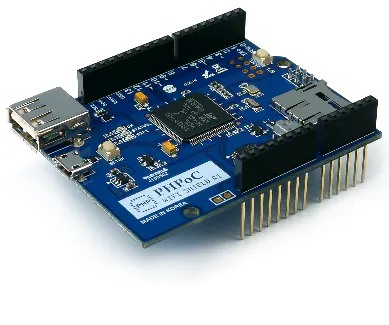# Arduino - Web-Based Gauge

This project shows how to monitor Arduino using a web-based gauge.

BeginnerFull instructions provided6,077

## Things used in this project

### Hardware componentsArduino UNO & Genuino UNO
×1PHPoC WiFi Shield for Arduino
×1
 Rotary Angle Sensor
×1UTSOURCE Electronic Parts
×1

## Code

### Arduino Code

Arduino
```#include "SPI.h"
#include "Phpoc.h"

PhpocServer server(80);
int sensorPin = A0;
float sensorValue = 0;  // variable to store the value coming from the sensor
int lastAngle = 0;

void setup() {
Serial.begin(9600);
while(!Serial)
;

Phpoc.begin(PF_LOG_SPI | PF_LOG_NET);

server.beginWebSocket("gauge");

Serial.println(Phpoc.localIP());
}

void loop() {
// when the client sends the first byte, say hello:
PhpocClient client = server.available();

if (client) {
sensorValue = 0;
for(int i = 0; i < 100; i++)

sensorValue /= 100;

int angle = (int)(sensorValue / 1024.0 * 280);

if(abs(angle - lastAngle) > 1){
String txtMsg = String(angle) + "\r\n";
char buf[txtMsg.length()];
txtMsg.toCharArray(buf, txtMsg.length());
server.write(buf, txtMsg.length());
Serial.println(txtMsg);

lastAngle = angle;
//delay(300);
}
}
}
```

### User Interface (remote_gauge.php)

PHP
```<!DOCTYPE html>
<html>
<title>PHPoC Shield / <?echo system("uname -i")?></title>
<meta name="viewport" content="width=device-width, initial-scale=0.7">
<style> body { text-align: center; } </style>
<script>
var canvas_width = 450, canvas_height = 450;
var pivot_x = canvas_width/2, pivot_y = canvas_height/2;
var hand_radius = 95, hand_max_angle = 280;
var ws;

function init()
{
var gage = document.getElementById("gage_01");
var ctx = gage.getContext("2d");

gage.width = canvas_width;
gage.height = canvas_height;

ctx.translate(pivot_x, pivot_y);
ctx.rotate(130 / 180 * Math.PI);

update_view(0);
}
function connect_onclick()
{
if(ws == null)
{
ws = new WebSocket("ws://<?echo _SERVER("HTTP_HOST")?>/gauge", "text.phpoc");
document.getElementById("ws_state").innerHTML = "CONNECTING";
ws.onopen = ws_onopen;
ws.onclose = ws_onclose;
ws.onmessage = ws_onmessage;

ws.onmessage = ws_onmessage;
}
else
ws.close();
}
function ws_onopen()
{
document.getElementById("ws_state").innerHTML = "<font color='blue'>CONNECTED</font>";
document.getElementById("bt_connect").innerHTML = "Disconnect";
ws.send("B\r\n");
}
function ws_onclose()
{
document.getElementById("ws_state").innerHTML = "<font color='gray'>CLOSED</font>";
document.getElementById("bt_connect").innerHTML = "Connect";
ws.onopen = null;
ws.onclose = null;
ws.onmessage = null;
ws = null;
}
function ws_onmessage(e_msg)
{
e_msg = e_msg || window.event; // MessageEvent

var angle = parseInt(e_msg.data);
console.log(angle);

update_view(angle);
}
function update_view(angle)
{
var gage = document.getElementById("gage_01");
var ctx = gage.getContext("2d");
var text;

if((angle < 0) || (angle > hand_max_angle))
return;

ctx.fillStyle = "#808080";
ctx.beginPath();
ctx.arc(0, 0, 215, 0, 2* Math.PI);
ctx.fill();

ctx.fillStyle = "#808080";
ctx.strokeStyle = "#bfbfbf";
ctx.lineWidth = 3;
ctx.beginPath();
ctx.arc(0, 0, 200, 0, 2* Math.PI);
ctx.fill();
ctx.stroke();

ctx.fillStyle = "#383330";
ctx.beginPath();
ctx.arc(0, 0, 180, 0, 2* Math.PI);
ctx.fill();

ctx.fillStyle = "black";
ctx.beginPath();
ctx.arc(0, 0, 165, 0, 2* Math.PI);
ctx.fill();

ctx.strokeStyle = "white";
ctx.fillStyle = "white";
ctx.save();

ctx.lineWidth = 10;
for(var i = 0; i < 5; i++)
{
ctx.beginPath();
ctx.stroke();
ctx.rotate(70 / 180 * Math.PI);
}
ctx.restore();
ctx.save();
ctx.rotate(35 / 180 * Math.PI);
ctx.lineWidth = 8;
for(var i = 0; i < 4; i++)
{
ctx.beginPath();
ctx.stroke();
ctx.rotate(70 / 180 * Math.PI);
}
ctx.restore();
ctx.save();
ctx.rotate( 17.5 / 180 * Math.PI);
ctx.lineWidth = 7;
for(var i = 0; i < 8; i++)
{
ctx.beginPath();
ctx.stroke();
ctx.rotate(35 / 180 * Math.PI);
}

ctx.restore();
ctx.lineCap = "round";
ctx.lineWidth = 7;

ctx.rotate(angle / 180 * Math.PI);
ctx.beginPath();
ctx.lineTo(0, 0);
ctx.stroke();
ctx.rotate(-angle / 180 * Math.PI);

ctx.beginPath();
ctx.arc(0, 0, 10, 0, 2* Math.PI);
ctx.fill();

ctx.rotate(-130 / 180 * Math.PI);
ctx.font = "24px Arial";
ctx.textAlign = "center";
ctx.fillText(angle + "°", 0, 50);
ctx.rotate(130 / 180 * Math.PI);
}
</script>
<body>

<h2>
Arduino - Dynamic Gauge<br>

<canvas id="gage_01"></canvas>

<p>
WebSocket : <span id="ws_state">null</span><br>
</p>
<button id="bt_connect" type="button" onclick="connect_onclick();">Connect</button>
</h2>

</body>
</html>
```

## Credits

### IoT_lover

11 projects • 142 followers